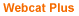### このページのリンク## ＜図書＞Cambridge mathematical library

出版者 Cambridge ; New York : Cambridge University Press und

### 子書誌情報を非表示

 1 The theory of partitions / George E. Andrews : pbk. - Cambridge : Cambridge University Press , 1998, c1984 2 Trigonometric series / A. Zygmund v. 1 and 2 combined : pbk. - 3rd ed. - Cambridge : Cambridge University Press , 2002 3 Abelian functions : Abel's theorem and the allied theory of theta functions / H.F. Baker : pbk. - Cambridge : Cambridge University Press , 1995 4 Methods of algebraic geometry / by W.V.D. Hodge and D. Pedoe 1 : pbk,2 : pbk,3 : pbk. - Cambridge : Cambridge University Press , 1994 5 An introduction to harmonic analysis / Yitzhak Katznelson : hard,: pbk.. - 3rd ed. - Cambridge, UK ; New York : Cambridge University Press , c2004 6 The geometry of moduli spaces of sheaves / Daniel Huybrechts and Manfred Lehn : pbk. - 2nd ed. - Cambridge ; New York : Cambridge University Press , 2010 7 Smooth compactifications of locally symmetric varieties / Avner Ash ... [et al.] ; with the collaboration of Peter Scholze : pbk. - 2nd ed. - Cambridge, UK ; New York : Cambridge University Press , 2010 8 A course of modern analysis : an introduction to the general theory of infinite processes and of analytic functions : with an account of the principal transcendental functions / by E. T. Whittaker and G. N. Watson : pbk. - 4th ed. - Cambridge : Cambridge University Press , 1996

### 書誌詳細を非表示

データ種別 図書 2000012506 BA0151012X